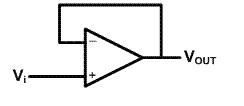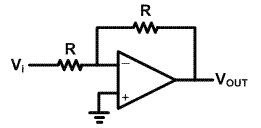# How to set the output voltage correctly in OTA design?

Status
Not open for further replies.

#### diemilio

##### Full Member level 6
Hello everyone!!

When designing an OTA, one of the most difficult taks is to set the output voltage to a desired value (when driving capacitive loads). I've heard that there are feedback approaches to set this output voltage. Could someone please explain me how can I do this???

Thanks,

diemilio

output offset values

Hi

If you have a single ended one, and you want to use the OTA in a negative feedback configuration, then this configuration itself can set the output voltage (should be design for suitable qualification). But if you have a fully differential configuration, you need a CMFB (common-mode feedback) circuit that its structure is depending on your OTA architecture..

re output offset

Is a single ended OTA.

What I don't understand is how feedback would set the output offset value to Vdd/2

Re: output offset

There are many architectures for applying negative feedback to an OTA. I explain you two of simplest (working for special applications). The first one is the simple negative feedback with the architecture below:In this case as you know the output voltage follows the input one (acting as a voltage buffer) according to the relationship below:

Vout = Av(Vp – Vn) => (Vp – Vn) = Vout/Av

For Av with large enough value Vout/Av app. equal to zero and so Vp app. equal to Vn = Vout.

So in this case the output voltage have the common-mode value equal to the common-mode of input signal and so the OTA should design for equal input and output common mode voltages.

The next common architecture is as follows:In this case with equal resistances, the circuit is also acting as buffer and the input and output common-mode voltages are equal. If you need different input and output common-mode voltages, you must choose suitable values for resistances, and you may need an additional circuitry for setting the OTA input common-mode voltage.

Points: 2